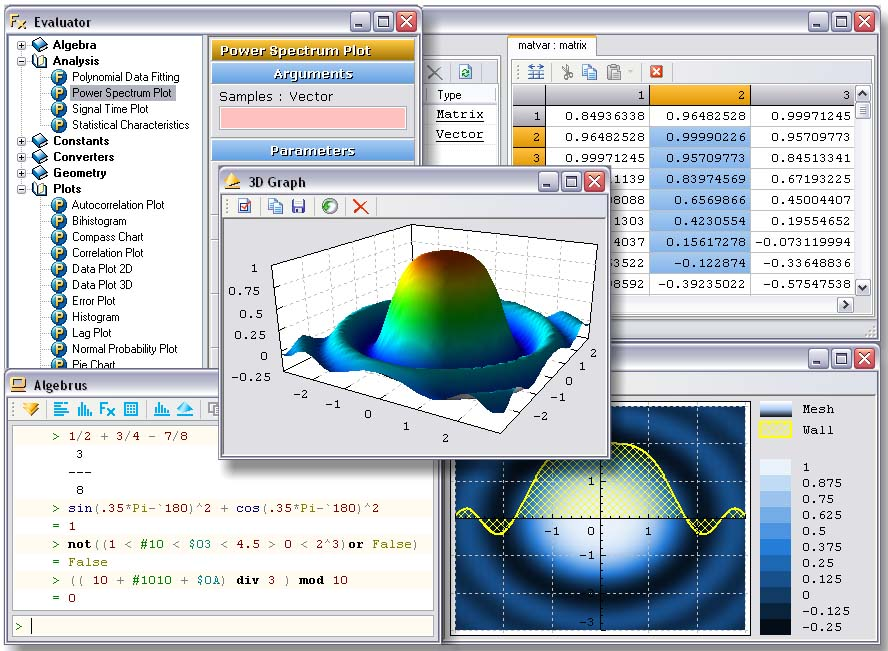# Algebrus 3.1

Program Info

Last Update: 2013-09-20

Release Status: Major Update

Size: 2.82 MB

Publisher: Astrise Corporation

OS Support: Win98, WinME, WinNT 4.x, Windows2000, WinXP

Category:

Rating
8Publisher's Description

P

owerful and extensible computer system for scientific and technical calculations. No matter if you are a school student or recognized scientist, Algebrus serves your needs. It is easy to use and interactive means of solving problem in various areas of expertise. Console based scientific calculator, 2D and 3D graphs plotter, differential, algebraic and polynomial equation solver, unit converter, physical constants reference, statistical analysis, data fitting, programming environment, and many more. Or just simple “evaluate-while-typing” calculator for your everyday arithmetics. If it doesn’t have something needed, you can use built-in integrated syntax-highlighted XPascal programming environment to create your own routines and libraries, define your own types and operators.

- Calculations and programming with vectors, matrices, complex numbers, fractions, quaternions, and polynomials
- 2D and 3D graphic plots and charts of functions and data
- Lines, scattered data, vector fields, contour plots, heights map, and meshes
- Parametric lines, histograms, error plots, spectrums, bar and pie charts
- Ordinary differential equations solving
- Linear algebraic equation system solving
- Statistical data analysis, data interpolation and fitting, Fourier and spectrum analysis
- Local and global multi-variable optimization
- Solving polynomial equations and finding polynomial extremums
- More than 250 built-in mathematical and analysis routines
- Data import-export to and from files, clipboard, serial ports
- More than 100 fundamental physical constants
- Calculation of different geometrical figures
- Converting to and from many dimensions of 20 different physical quantities
- Interactive user interface for function evaluation and graph plotting